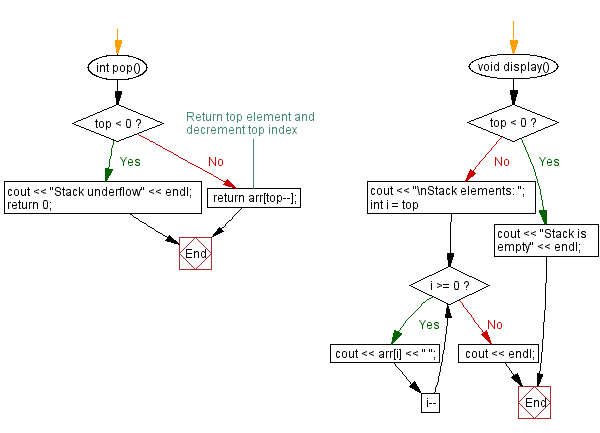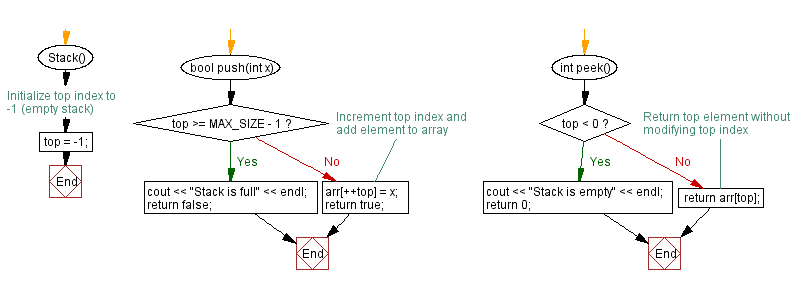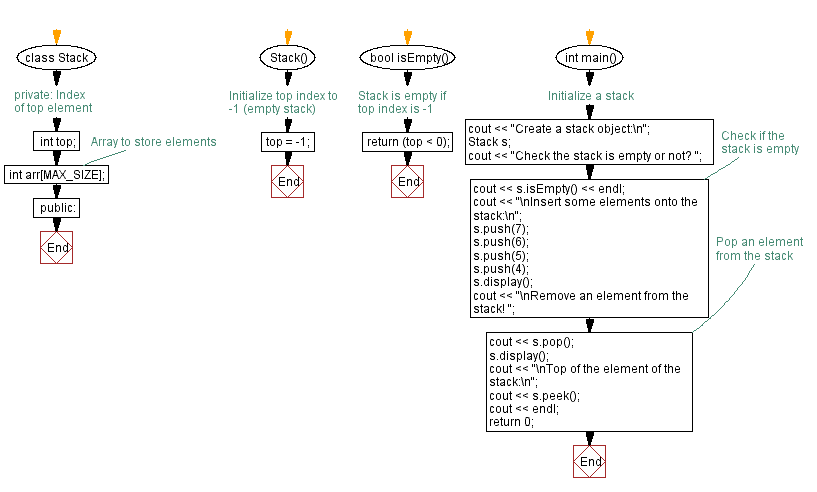﻿ C++ Implement a stack using an array with push, pop operations

# C++ Stack Exercises: Implement a stack using an array with push, pop operations

## C++ Stack: Exercise-1 with Solution

Write a C++ program to implement a stack using an array with push and pop operations. Find the top element of the stack and check if the stack is empty or not.

Test Data:
Create a stack object:
Check the stack is empty or not? 1
Insert some elements onto the stack:
Stack elements: 4 5 6 7
Remove an element from the stack! 4
Stack elements: 5 6 7

Sample Solution:

C++ Code:

``````#include <iostream>
using namespace std;
#define MAX_SIZE 1000 // Maximum size of stack
class Stack {
private:
int top; // Index of top element
int arr[MAX_SIZE]; // Array to store elements
public:
Stack() {
top = -1; // Initialize top index to -1 (empty stack)
}

bool push(int x) {
if (top >= MAX_SIZE - 1) {
cout << "Stack is full" << endl;
return false;
}
arr[++top] = x; // Increment top index and add element to array
return true;
}

int pop() {
if (top < 0) {
cout << "Stack underflow" << endl;
return 0;
}
}
int peek() {
if (top < 0) {
cout << "Stack is empty" << endl;
return 0;
}
}
bool isEmpty() {
return (top < 0); // Stack is empty if top index is -1
}
void display() {
if (top < 0) {
cout << "Stack is empty" << endl;
return;
}
cout << "\nStack elements: ";
for (int i = top; i >= 0; i--)
cout << arr[i] << " ";
cout << endl;
}
};
int main() {
//Initialize a stack
cout << "Create a stack object:\n";
Stack s;
cout << "Check the stack is empty or not? ";
cout << s.isEmpty() << endl; // Check if the stack is empty
cout << "\nInsert some elements onto the stack:\n";
s.push(7);
s.push(6);
s.push(5);
s.push(4);
s.display();
cout << "\nRemove an element from the stack! ";
cout << s.pop(); // Pop an element from the stack
s.display();
cout << "\nTop of the element of the stack:\n";
cout << s.peek();
cout << endl;
return 0;
}
``````

Sample Output:

```Create a stack object:
Check the stack is empty or not? 1

Insert some elements onto the stack:

Stack elements: 4 5 6 7

Remove an element from the stack! 4
Stack elements: 5 6 7

Top of the element of the stack:
5
```

Flowchart:CPP Code Editor: